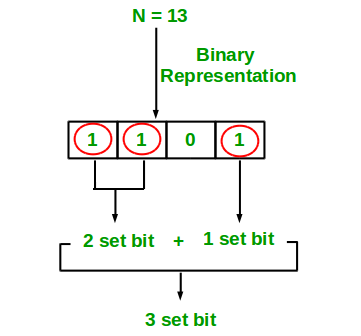# C# Program for Count set bits in an integer

Write an efficient program to count number of 1s in binary representation of an integer.

Examples :

```Input : n = 6
Output : 2
Binary representation of 6 is 110 and has 2 set bits

Input : n = 13
Output : 3
Binary representation of 11 is 1101 and has 3 set bits
```## Recommended: Please solve it on “PRACTICE ” first, before moving on to the solution.

1. Simple Method Loop through all bits in an integer, check if a bit is set and if it is then increment the set bit count. See below program.

## C#

 `// C# program to Count set ` `// bits in an integer ` `using` `System; ` ` `  `class` `GFG { ` `    ``// Function to get no of set ` `    ``// bits in binary representation ` `    ``// of positive integer n ` `    ``static` `int` `countSetBits(``int` `n) ` `    ``{ ` `        ``int` `count = 0; ` `        ``while` `(n > 0) { ` `            ``count += n & 1; ` `            ``n >>= 1; ` `        ``} ` `        ``return` `count; ` `    ``} ` ` `  `    ``// Driver Code ` `    ``public` `static` `void` `Main() ` `    ``{ ` `        ``int` `i = 9; ` `        ``Console.Write(countSetBits(i)); ` `    ``} ` `} ` ` `  `// This code is contributed by Sam007 `

Output:

```2
```

Recursive Approach :

## C#

 `// C# implementation of recursive ` `// approach to find the number of ` `// set bits in binary representation ` `// of positive integer n ` `using` `System; ` ` `  `class` `GFG { ` ` `  `    ``// recursive function ` `    ``// to count set bits ` `    ``public` `static` `int` `countSetBits(``int` `n) ` `    ``{ ` ` `  `        ``// base case ` `        ``if` `(n == 0) ` `            ``return` `0; ` ` `  `        ``else` ` `  `            ``// if last bit set ` `            ``// add 1 else add 0 ` `            ``return` `(n & 1) + countSetBits(n >> 1); ` `    ``} ` ` `  `    ``// Driver code ` `    ``static` `public` `void` `Main() ` `    ``{ ` ` `  `        ``// get value ` `        ``// from user ` `        ``int` `n = 9; ` ` `  `        ``// function calling ` `        ``Console.WriteLine(countSetBits(n)); ` `    ``} ` `} ` ` `  `// This code is contributed by aj_36 `

Output:

```2
```

Please refer complete article on Count set bits in an integer for more details!

My Personal Notes arrow_drop_up
Article Tags :

Be the First to upvote.

Please write to us at contribute@geeksforgeeks.org to report any issue with the above content.